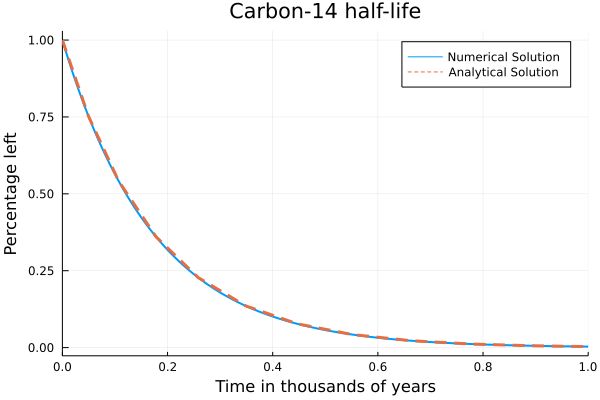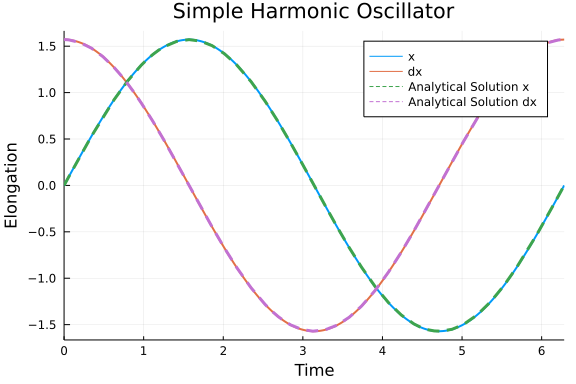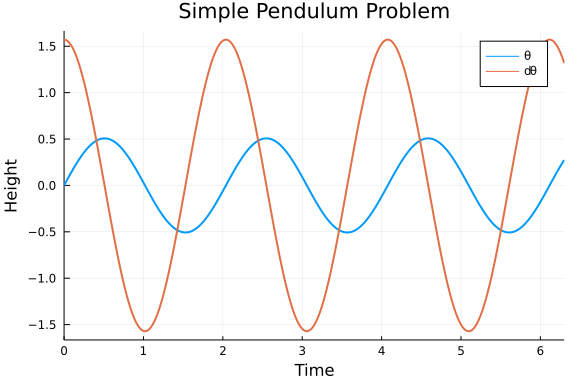# Classical Physics Models

##### Yingbo Ma, Chris Rackauckas

If you're getting some cold feet to jump in to DiffEq land, here are some handcrafted differential equations mini problems to hold your hand along the beginning of your journey.

## First order linear ODE

$f(t,u) = \frac{du}{dt}$

The Radioactive decay problem is the first order linear ODE problem of an exponential with a negative coefficient, which represents the half-life of the process in question. Should the coefficient be positive, this would represent a population growth equation.

using OrdinaryDiffEq, Plots
gr()

#Half-life of Carbon-14 is 5,730 years.
C₁ = 5.730

#Setup
u₀ = 1.0
tspan = (0.0, 1.0)

#Define the problem

#Pass to solver
sol = solve(prob,Tsit5())

#Plot
plot(sol,linewidth=2,title ="Carbon-14 half-life", xaxis = "Time in thousands of years", yaxis = "Percentage left", label = "Numerical Solution")
plot!(sol.t, t->exp(-C₁*t),lw=3,ls=:dash,label="Analytical Solution")## Second Order Linear ODE

#### Simple Harmonic Oscillator

Another classical example is the harmonic oscillator, given by

$\ddot{x} + \omega^2 x = 0$

with the known analytical solution

\begin{align*} x(t) &= A\cos(\omega t - \phi) \\ v(t) &= -A\omega\sin(\omega t - \phi), \end{align*}

where

$A = \sqrt{c_1 + c_2} \qquad\text{and}\qquad \tan \phi = \frac{c_2}{c_1}$

with $c_1, c_2$ constants determined by the initial conditions such that $c_1$ is the initial position and $\omega c_2$ is the initial velocity.

Instead of transforming this to a system of ODEs to solve with ODEProblem, we can use SecondOrderODEProblem as follows.

# Simple Harmonic Oscillator Problem
using OrdinaryDiffEq, Plots

#Parameters
ω = 1

#Initial Conditions
x₀ = [0.0]
dx₀ = [π/2]
tspan = (0.0, 2π)

ϕ = atan((dx₀/ω)/x₀)
A = √(x₀^2 + dx₀^2)

#Define the problem
function harmonicoscillator(ddu,du,u,ω,t)
ddu .= -ω^2 * u
end

#Pass to solvers
prob = SecondOrderODEProblem(harmonicoscillator, dx₀, x₀, tspan, ω)
sol = solve(prob, DPRKN6())

#Plot
plot(sol, vars=[2,1], linewidth=2, title ="Simple Harmonic Oscillator", xaxis = "Time", yaxis = "Elongation", label = ["x" "dx"])
plot!(t->A*cos(ω*t-ϕ), lw=3, ls=:dash, label="Analytical Solution x")
plot!(t->-A*ω*sin(ω*t-ϕ), lw=3, ls=:dash, label="Analytical Solution dx")Note that the order of the variables (and initial conditions) is dx, x. Thus, if we want the first series to be x, we have to flip the order with vars=[2,1].

## Second Order Non-linear ODE

#### Simple Pendulum

We will start by solving the pendulum problem. In the physics class, we often solve this problem by small angle approximation, i.e. $sin(\theta) \approx \theta$, because otherwise, we get an elliptic integral which doesn't have an analytic solution. The linearized form is

$\ddot{\theta} + \frac{g}{L}{\theta} = 0$

But we have numerical ODE solvers! Why not solve the real pendulum?

$\ddot{\theta} + \frac{g}{L}{\sin(\theta)} = 0$

Notice that now we have a second order ODE. In order to use the same method as above, we nee to transform it into a system of first order ODEs by employing the notation $d\theta = \dot{\theta}$.

\begin{align*} &\dot{\theta} = d{\theta} \\ &\dot{d\theta} = - \frac{g}{L}{\sin(\theta)} \end{align*}

# Simple Pendulum Problem
using OrdinaryDiffEq, Plots

#Constants
const g = 9.81
L = 1.0

#Initial Conditions
u₀ = [0,π/2]
tspan = (0.0,6.3)

#Define the problem
function simplependulum(du,u,p,t)
θ = u
dθ = u
du = dθ
du = -(g/L)*sin(θ)
end

#Pass to solvers
prob = ODEProblem(simplependulum, u₀, tspan)
sol = solve(prob,Tsit5())

#Plot
plot(sol,linewidth=2,title ="Simple Pendulum Problem", xaxis = "Time", yaxis = "Height", label = ["\\theta" "d\\theta"])So now we know that behaviour of the position versus time. However, it will be useful to us to look at the phase space of the pendulum, i.e., and representation of all possible states of the system in question (the pendulum) by looking at its velocity and position. Phase space analysis is ubiquitous in the analysis of dynamical systems, and thus we will provide a few facilities for it.

p = plot(sol,vars = (1,2), xlims = (-9,9), title = "Phase Space Plot", xaxis = "Velocity", yaxis = "Position", leg=false)
function phase_plot(prob, u0, p, tspan=2pi)
_prob = ODEProblem(prob.f,u0,(0.0,tspan))
sol = solve(_prob,Vern9()) # Use Vern9 solver for higher accuracy
plot!(p,sol,vars = (1,2), xlims = nothing, ylims = nothing)
end
for i in -4pi:pi/2:4π
for j in -4pi:pi/2:4π
phase_plot(prob, [j,i], p)
end
end
plot(p,xlims = (-9,9))